• ## MATLAB删除矩阵元素

千次阅读 2020-06-05 19:06:56
MATLAB删除矩阵元素
已知矩阵

a

a

，删去其中第

i

i

个元素，可通过如下命令：

a

(

i

)

=

[

]

a(i)=[]

代码如下：
a=[1 2 3;4 5 6];
a(1)=[];

运行结果：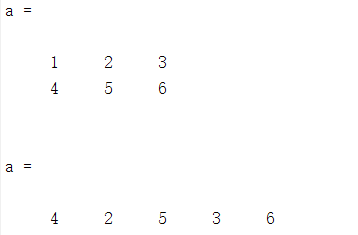需要注意的是执行删除操作后，矩阵自动重新排布成行向量。
展开全文• 矩阵中提取元素元素群 a = 1.0000 2.0000 3.0000 4.0000 5.0000 6.0000 7.0000 8.0000 9.0000 0 0 6.5000 5.0000 4.0000 3.0000 b=a([2,4],[1,3]) %提取矩阵a中2,4 两行的1,3 列数据 b = 4.00...
在矩阵中提取元素或元素群
a =
1.0000    2.0000    3.0000
4.0000    5.0000    6.0000
7.0000    8.0000    9.0000
0         0    6.5000
5.0000    4.0000    3.0000

b=a([2,4],[1,3]) %提取矩阵a中2,4 两行的1,3 列数据
b =
4.0000    6.0000
0    6.5000

用空矩阵 [ ] 在矩阵中删除行或列 a([2,4,5],:)=[] a =
 1     2     3
7     8     9

矩阵的转置
a’
ans =
 1     7
2     8
3     9

展开全文• deleteRC.m 自己写的代码，还算好用，可以一次性删除任意行或列的数据 删除矩阵X中index指定的行或列，type='r'表示行，type='c'表示列
• RANDELEMENT(ARRAY,N) 返回一行 N 个元素，这些元素是从 ARRAY 中随机选择（和替换）的。 [可能有一些工具箱功能可以做一些非常相似的事情。] 例子： randelement([0:9],5) 可能会返回 [4 8 8 5 2] randelement...
• 一、矩阵元素的引用方式 1、通过下标来引用矩阵的元素，下标必须为正整数，且要用圆括号括起来 ...在MATLAB中，矩阵元素按列存储，即首先存储矩阵的第一列元素，然后存储第二列元素，…，一直到矩阵的
一、矩阵元素的引用方式
1、通过下标来引用矩阵的元素，下标必须为正整数，且要用圆括号括起来
例子（1）： A(3,2)表示A矩阵第3行第2列的元素。 如果要给这个元素赋值200。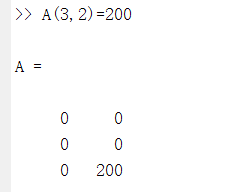注意：这只是改变了A（3,2）这个元素的值，并不会影响到其他的元素
例子（2）：
当给出的行列下标超出原来矩阵的行数和列数的时候，在matlab中将自动扩展原来的矩阵，并将扩展后没有赋值的矩阵元素，置为0.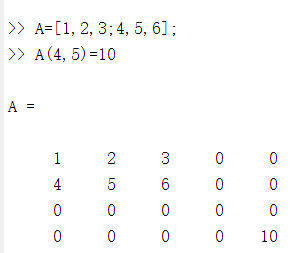2、通过序号来引用
在MATLAB中，矩阵元素按列存储，即首先存储矩阵的第一列元素，然后存储第二列元素，…，一直到矩阵的最后一列元素。
矩阵元素的序号就是矩阵元素在内存中的排列顺序。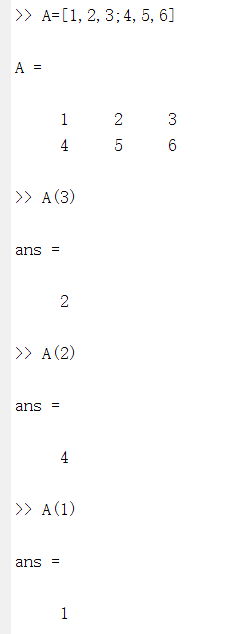序号与下标是一一对应的，以m×n矩阵A为例，矩阵元素A(i,j)的序号为(j-1)×m+i

矩阵元素的序号与下标可以利用sub2ind和ind2sub函数实现相互转换。 sub2ind函数：将矩阵中指定元素的行、列下标转换成存储的序号。调用格式为： D=sub2ind(S,I,J) D：表示对应下标元素的序号，它的行列数与I和J相同 S：表示要转换的的矩阵的行数和列数，是由行数和列数组成的向量，通常用size函数来获取 I：是要转化矩阵元素的行下标 J：是要转换矩阵的列下标 如果I和J是矩阵，表示要将矩阵中的多个元素的行列下标转换成存储的序号，及I和J的行列数必须相同。sub2ind函数中第一个参数通过size函数来获取，得到矩阵A的行数和列数，是一个包含两个元素的向量，分别为A矩阵的行数2和列数3。第二个和第三个参数都是用矩阵表示的，两个矩阵对应的元素代表矩阵A 中的某个元素的行下标和列下标，由调用结果可知：行下标为1，列下标为1的元素，在矩阵中是序号为1的；行下标为2列下标为1的元素在矩阵中序号为2；行下标为2列下标为3的元素在矩阵中序号为6；行下标为2列下标为2的元素在矩阵中序号为4.

ind2sub函数：将把矩阵元素的序号转换成对应的下标，其调用格式为：
[I,J]=ind2sub(S,D)
S：表示要转换的矩阵对的行数和列数，是由行数和列数组成的有两个元素的向量 D：序号，函数返回值为序号所对应元素的行下标和列下标 I：对应元素的行下标 j ；对应元素的列下标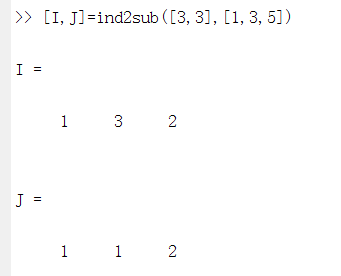[3,3]表示是一个3行3列的矩阵 [1,3,5]表示要求一个3行3列矩阵的第一个，第三个，第五个元素的下标 结果表明：第一个，第三个，第五个元素的下标分别为(1,1),(3,1),(2,2)。
二、利用冒号表达式获得子矩阵
子矩阵是指由矩阵中的一部分元素构成的矩阵。 A(i,:) 第i行的全部元素 A(:,j) 第j列的全部元素 A(i:i+m,k:k+m) 第i～i+m行内且在第k～k+m列中的所有元素 A(i:i+m,:) 第i～i+m行的全部元素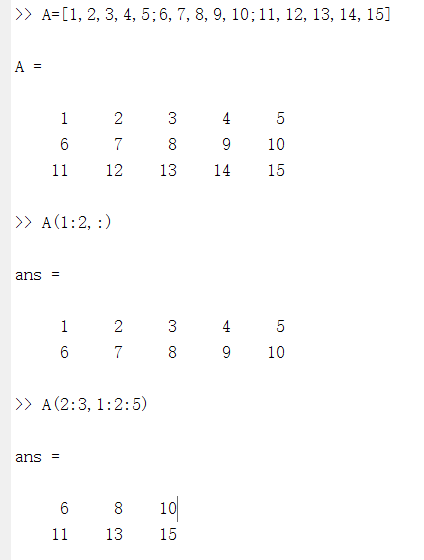end运算符：表示某一维的末尾元素下标。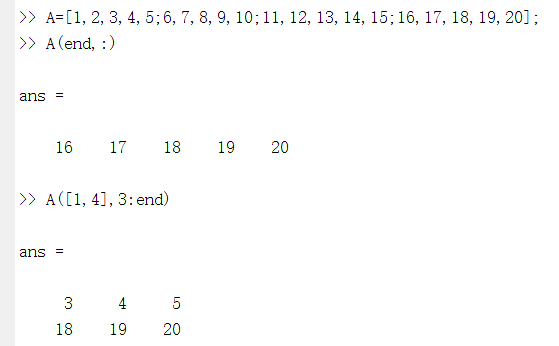A(end,:)表示应用矩阵A 的最后一行的元素。 A([1,4],3:end)表示应用第一行和第四行的从第三列到最后一列的元素。
三、利用空矩阵删除矩阵的元素
空矩阵是指没有任何元素的矩阵。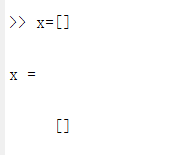X是一个空矩阵。如果要将某些元素从一个矩阵中删除，直接将这些元素置为空矩阵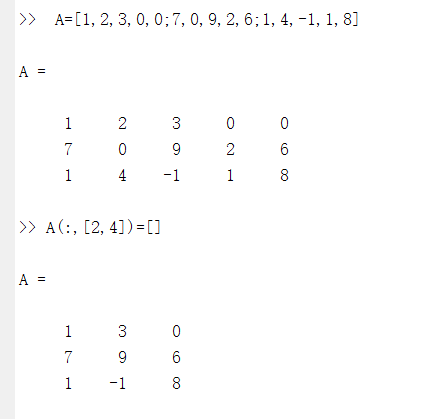A(:,[2,4])=[]表示赋空矩阵，删除第二列和第四列元素。
四、改变矩阵的形状
reshape(A,m,n)：在矩阵总元素保持不变的前提下，将矩阵A重新排成m×n的二维矩阵。 注意：reshape函数只是改变原矩阵的行数和列数，但并不改变原矩阵元素个数及其存储顺序。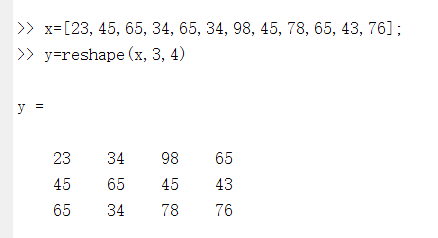y=reshape(x,3,4)表示调用reshape函数，利用向量x建立3行4列的矩阵赋给y 矩阵x为1行12列的矩阵 矩阵y为2行4列的矩阵

A(: ) ：将矩阵A的每一列元素堆叠起来，成为一个列向量。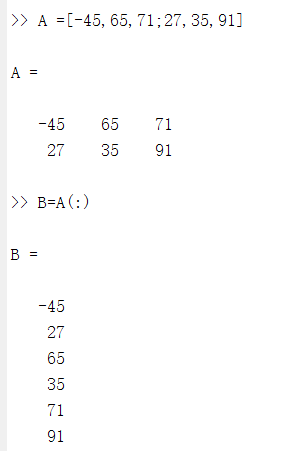可知A(:)等价于reshape(A,6,1)。
展开全文• Matlab删除矩阵中的制定元素 >> a=[1,2,3,4] a = 1 2 3 4 >> a==2 ans = 1×4 logical 数组 0 1 0 0 >> a(a==2)=[] a = 1 3 4 a == 2: 构建索引 a(a==2)=[ ]:将...
Matlab删除矩阵中的制定元素
>> a=[1,2,3,4]

a =

1     2     3     4

>> a==2

ans =

1×4 logical 数组

0   1   0   0

>> a(a==2)=[]

a =

1     3     4

a == 2: 构建索引 a(a==2)=[ ]:将指定索引的元素变为空[ ]
展开全文• 有一个矩阵, 举例如下: A = 6 11 17 1 1 1 3 5 6 6 11 15 1 3 6 ...目前网上的教程都是如何删除元素相同的行, 那么如果我们想删除元素相同的一行, 比如1 1 1该怎么办呢? 我们可以换个思路: 把
• 想去掉矩阵里面重复的元素，或者计算每个不同元素出现的次数。发现了matlab的集合运算函数，准备总结一下。 集合运算操作有交、并等操作，这篇主要介绍unique这个函数的一些用法 1、去掉矩阵A中重复的行 2、元素的...
• all函数：检测矩阵中是否全为零元素 扩充：any函数，检测矩阵中是否有非零元素，有返回1，无返回0 **vector = all(array, 1)**返回一个行向量，可以认为all(A, 1)等价于all(A) **vector = all(array, 2)**返回一个列...
• Matlab(5)——矩阵元素的引用 文章目录Matlab(5)——矩阵元素的引用一、矩阵元素的引用方式1.通过下标来引用矩阵的元素2.通过序号来引用矩阵的元素二、利用冒号表达式获得子矩阵三、利用空矩阵来删除矩阵的元素四、...线性代数
• 如果给出的下标大于矩阵的行数或列数，那么matlab将会自动扩展原来的矩阵，并将扩展后未进行赋值的元素置为0。 （2）通过序号 红色标识为序号 下标与索引相互转换 sub2ind函数 ind2sub函数 下列语句表示： 求在...
• ## MATLAB矩阵元素的提取和替换

万次阅读 多人点赞 2017-05-17 10:48:53
1. 提取1 . 单个元素的提取a=[1,2,3;3,4,5]a = 1 2 3 3 4 5b=a(1,2)b = 2 2 . 提取矩阵中某一行的元素a=[1,2,3;3,4,5],运行后：a = 1 2 3 3 4 5b=a(1,:)b = 1 2 33 . 提
• 矩阵元素的引用方式： （1）通过下标来引用矩阵的元素 A(3,2)表示A矩阵第3行第2列的元素。 A(3,2)=200 先创建矩阵A=[1,2,3;4,5,6];再输入A(4,5)=10 得到： A = 1 2 3 0 0 4 5 6 0 0 0 0 0 0 0 0 0 0 0 10 （2）...
• MATLAB基础知识1 MATLAB矩阵的表示1.1矩阵建立1.2结构矩阵和单元矩阵2矩阵元素引用2.1引用方式2.2冒号表达式获得子矩阵2.3空矩阵删除矩阵的元素2.4改变矩阵的形状3 MATLAB基本运算3.1算数运算3.2关系运算3.3逻辑运算...
• 1，知道该行的行号 删除第2行 a = [1 1; 2 2;...2，不知道行号，删除指定的元素 a = [1 1; 2 2; 3 3; 4 4; 2 2]; b = [3 3]; c = setdiff(a,b,'row','stable'); 得到：c = [1 1; 2 2; 4 4; 2 2]; ...setdiff
• 向量： a=[1,0,2,3,0,4,5] a = 1 0 2 3 0 4 5 a(find(a==0))=[] a = 1 2 3 4 5 矩阵： a=[0 0;1 2;0 0;3 4] a = 0 0 1 2 0 0 3 ...
• 利用空矩阵删除矩阵元素矩阵是指没用任何元素矩阵。即 >> X=[] X = [] 如果要将矩阵中的元素删除，我们就将矩阵中的元素置为空就可以了。例如： >> clear all %创建3行5列矩阵A >> ...
• Matlab2016中，输入矩阵A=[1 2 3;4 5 6;7 8 9];只取矩阵中第一行的元素A1=A(1,:); 若要删除第一行的元素则A(1,:)=[] <textarea readonly="readonly" name="code" class="c++"> >> A=[1 2 3;4 5 6;7 8 ...
• 在用matlab编程进行GNSS粗差提取时，想实现对某矩阵删除指定行（指定列）的命令，下文中描述的均为行。通过百度，先明白对于一行数据而言，删除指定位置的元素的原理。主要思路是先提取要删除的位置，再对该位置数据...
• MATLAB中，矩阵元素是按列存储的，即首先存储矩阵第一列元素，然后存储第二列元素，……，一直到矩阵的最后一列。 矩阵元素的序号就是矩阵元素在内存中的排列顺序。 序号与下标是一一对应的，以m*n矩阵A为例，矩阵...
• ## Matlab删除重复元素

万次阅读 2018-10-18 16:01:52
Matlab 删除重复元素删除重复行 函数：unique b = unique(A) b = unique(A,'rows') [b,m,n] = unique(...) 描述 1、b = unique(A) 返回的是和A中一样的值，但是没有重复元素。产生的结果向量按升序排序。A可以...
• ## MATLAB中矩阵的逻辑索引方法

千次阅读 多人点赞 2019-05-27 11:02:29
MATLAB中对矩阵元素的索引，包括常用的下标法和巧妙的逻辑索引，逻辑索引是用逻辑0或1去判断对应位置的元素是否被选择，所以矩阵和逻辑阵维数必须一致；是不是可能有点不明白，贴个例子你就懂啦： >>A=[-1 1;...逻辑索引
• % 邻接矩阵的添加与删除 s = [1,2,3]; t = [2,3,1]; G = graph(s,t) % 添加 G = G.addedge(2,4) % 删除 G = G.rmedge(2,4)
• G=[1,2,3;4,5,6;7,8,9];删除第三行G(3,:) = []; 删除第3列G(:,3) = [];
• ## matlab中矩阵删除一行或一列的方法

万次阅读 多人点赞 2017-11-23 22:06:54
• (2)通过序号来引用，在MATLAB矩阵元素按列存储。序号与下标是一一对应的，以m*n的矩阵A为例，矩阵A(i，j)的序号就是(j-1)*m+isub2ind函数：将矩阵中指定元素的行、列下标转换成存储的序号。调用格式为D=sub2ind(S,...
• MATLAB删除矩阵的某一列或某一行删除某一行例子删除某一列例子 删除某一行 b([列数],:) = []; // b为矩阵名 例子 删除第一行 删除第三行 删除某一列 b(:,[列数]) = []; // b为矩阵名 例子 删除第...
• 本期视频时长约120分钟，通过海量的实例，通俗易懂地讲解了MATLAB变量，数组和矩阵，数值类型，矩阵的创建，矩阵元素的提取、赋值和删除操作，矩阵的合并，矩阵的四则运算等重要内容，帮助零基础学习MATLAB的同学，......

# matlab删除矩阵元素matlab 订阅